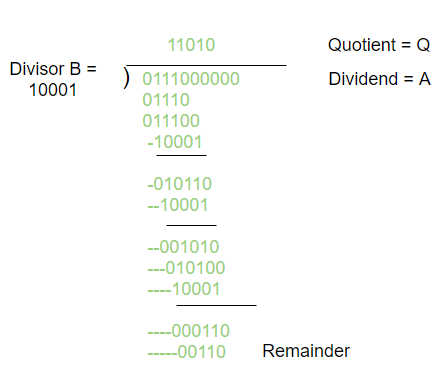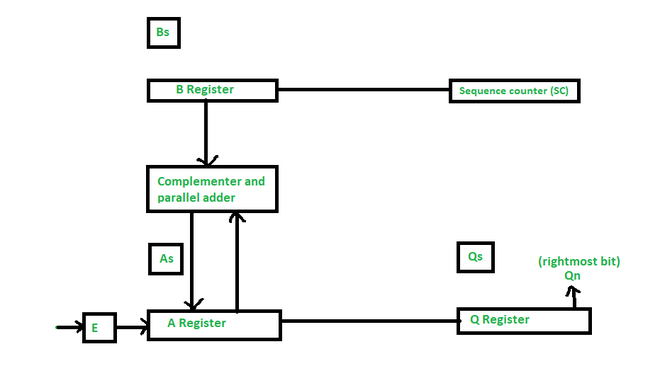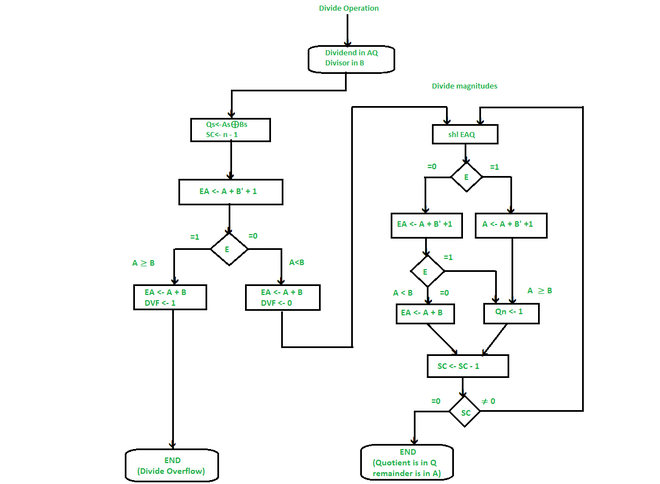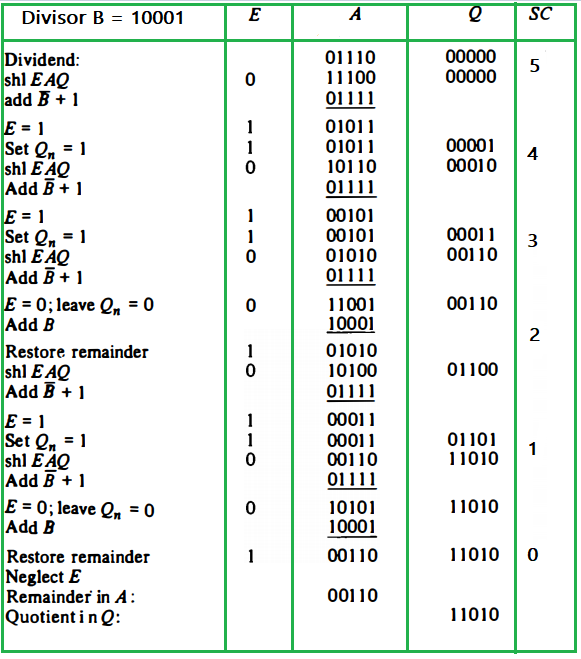# Division Algorithm in Signed Magnitude Representation

• Difficulty Level : Hard
• Last Updated : 11 Jan, 2021

The Division of two fixed-point binary numbers in the signed-magnitude representation is done by the cycle of successive compare, shift, and subtract operations.

The binary division is easier than the decimal division because the quotient digit is either 0 or 1. Also, there is no need to estimate how many times the dividend or partial remainders adjust to the divisor.

Attention reader! Don’t stop learning now. Get hold of all the important CS Theory concepts for SDE interviews with the CS Theory Course at a student-friendly price and become industry ready.Hardware Implementation :The hardware implementation in the division operation is identical to that required for multiplication and consists of the following components –

• Here, Registers B is used to store divisor and the double-length dividend is stored in registers A and Q
• The information for the relative magnitude is given in E.
• Sequence Counter register (SC) is used to store the number of bits in the dividend.

Flowchart of Division :• Initially, the dividend is in A & Q and the divisor is in B.

• Sign of result is transferred into Q, to be the part of quotient. Then a constant is set into the SC to specify the number of bits in the quotient.

• Since an operand must be saved with its sign, one bit of the word will be inhabited by the sign, and the magnitude will be composed of n -1 bits.

• The condition of divide-overflow is checked by subtracting the divisor in B from the half of bits of the dividend stored in A. If A ≥ B, DVF is set and the operation is terminated before time. If A < B, no overflow condition occurs and so the value of the dividend is reinstated by adding B to A.

• The division of the magnitudes starts by shl dividend in AQ to left in the high-order bit shifted into E.
(Note – If shifted a bit into E is equal to 1, and we know that EA > B as EA comprises a 1 followed by n -1 bits whereas B comprises only n -1 bits). In this case, B must be subtracted from EA, and 1 should insert into Q, for the quotient bit.

• If the shift-left operation (shl) inserts a 0 into E, the divisor is subtracted by adding its 2’s complement value and the carry is moved into E. If E = 1, it means that A ≥ B; thus, Q, is set to 1. If E = 0, it means that A < B and the original number is reimposed by adding B into A.

• Now, this process is repeated with register A containing the partial remainder.

Example of a binary division using digital hardware :

Divisor B = 10001, Dividend A = 0111000000Final Remainder : 00110

My Personal Notes arrow_drop_up
Recommended Articles
Page :